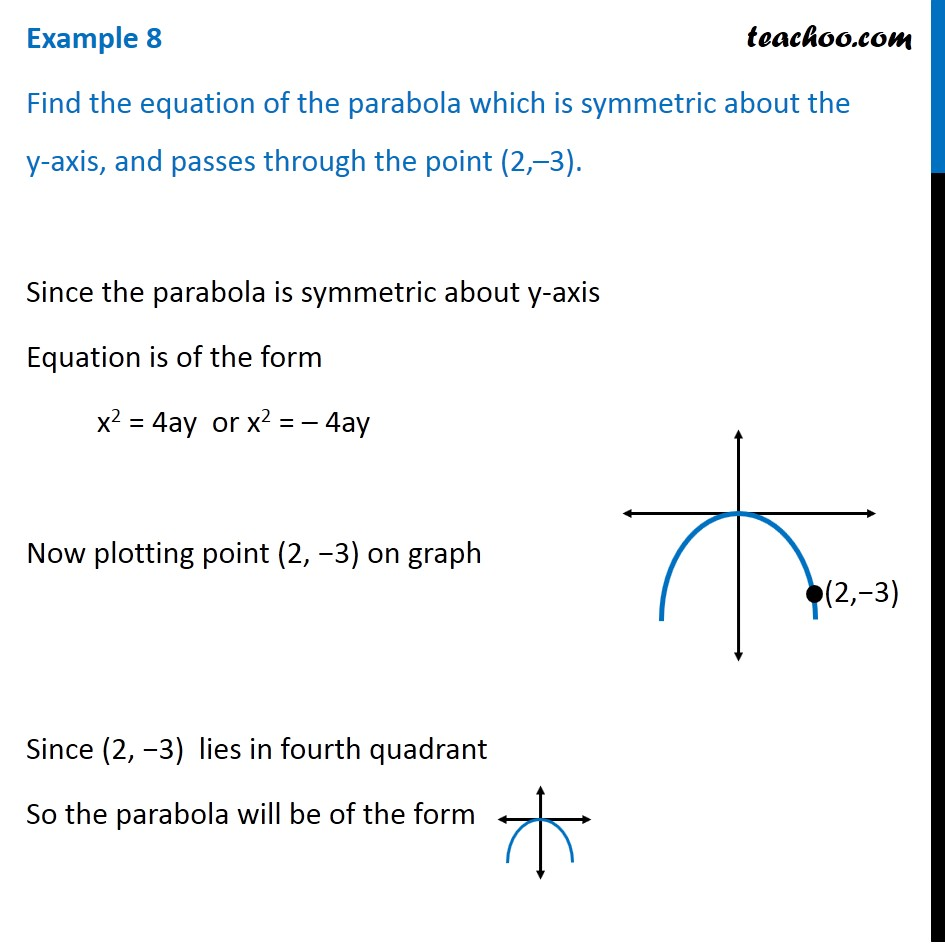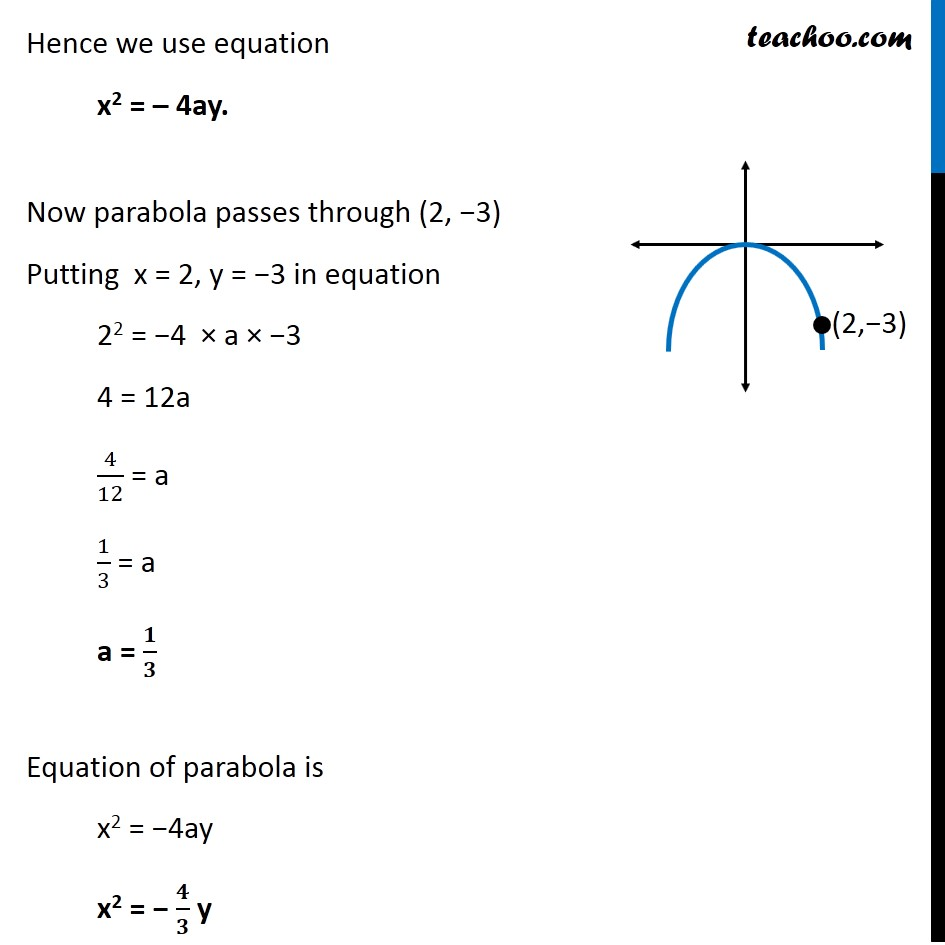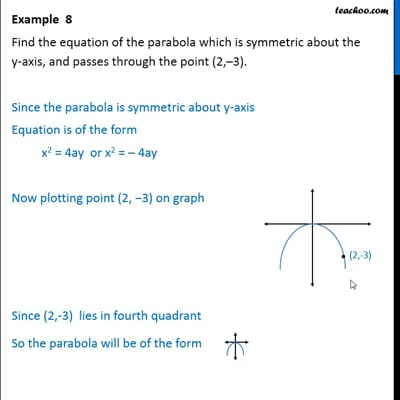Examples

Chapter 11 Class 11 Conic Sections (Term 2)
Serial order wiseThis video is only available for Teachoo black users

### Transcript

Example 8 Find the equation of the parabola which is symmetric about the y-axis, and passes through the point (2,–3). Since the parabola is symmetric about y-axis Equation is of the form x2 = 4ay or x2 = – 4ay Now plotting point (2, −3) on graph Since (2, −3) lies in fourth quadrant So the parabola will be of the form Hence we use equation x2 = – 4ay. Now parabola passes through (2, −3) Putting x = 2, y = −3 in equation 22 = −4 × a × −3 4 = 12a 4/12 = a 1/3 = a a = 𝟏/𝟑 Equation of parabola is x2 = −4ay x2 = − 𝟒/𝟑 y